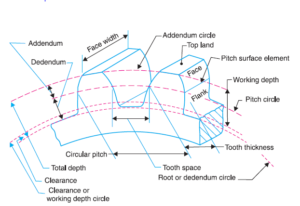## Gear Ratio: Definition, Formula, Advantages, Uses

Gear Ratio The gear ratio of a gear train is the ratio of the angular velocity of the input gear to the angular velocity of the output gear, also known as the speed ratio of the gear train. The gear ratio can be computed directly from the numbers of teeth of the...## Linear Programming Problems Definition

In this post, we try to understand the basics of Linear Programming Problems. We get to know the definition, the terms used, and then understanding the terms, their definition, and role in LPP. Introduction With Linear Programming, a technique, we approach towards...
error: Content is protected !!